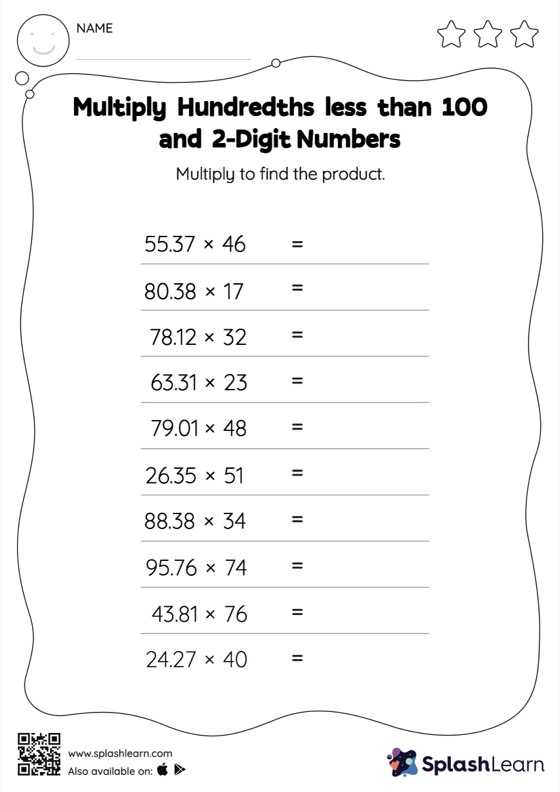# Multiply Hundredths less than 100 and 2-Digit Numbers: Horizontal Multiplication Worksheet

Home > Multiply Hundredths less than 100 and 2-Digit Numbers: Horizontal MultiplicationThis worksheet invites students to multiply hundredths less than 100 and 2-digit numbers, which helps them become proficient in multiplication. This worksheet is about practicing with the horizontal format in which numbers are written side by side. To develop flexibility with numbers and operations, students need to have enough practice in this format and not just rely on the vertical/column method.